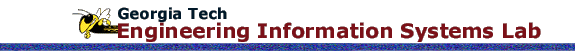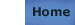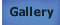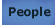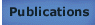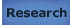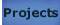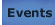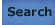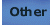# Evaluation of Move-Limits Using the Two-Point Exponential Approximation in Structural Optimization

## Reference

Fadel, G.M.; Cimtalay, S.(1993) Evaluation of Move-Limits Using the Two-Point Exponential Approximation in Structural Optimization, American Society of Mechanical Engineers, AerospaceDivision (AD), Structures & Controls Optimization ASME Aerospace Division, ASME, New York, v. 38, 83-92.

## Abstract

Move-limits in structural optimization are used to control the validity of approximations and to minimize the risk of getting trapped outside the feasible domain. The Two Point Exponential Approximation Method was introduced by Fadel et al. [Fadel, 19901 , and tested on structural optimization problems with stress and displacement constraints as well as on problems with frequency constra ints (Sareen, 1991 1. The method, which consists in correcting Taylor series approximations using previous design history, is used in the present paper to automatically determine move-limits. Move-limits are the allowable changes in design variables duri ng the optimization of the approximate problem. The exponents, computed in the Two Point Exponential Approximation by matching slopes between two design iterations, are used as a measure of non-hnearity of the objective and constraints with respect to ea ch of the design variables. The relationship between the move-limits and the exponents is derived and individual move-limits are computed and applied to each design variable. The method is applied to two classical structural optimization examples. It pro vides the engineer with more fleidbihty when choosing the move-limits and typically results in faster convergence.

## Documents

Manuscript: pdf (1162K)